# Charts: The Essentials

If you're looking to display your data with a more visual-driven focus, then you should consider using Chart views.

When creating a chart, you should ask yourself three different questions:

• What chart display type should you use?
• What values do you want on the X and Y axes? This would be determined by the orientation of your data set.
• How many individual series on the chart do you want to draw? This would be determined by the number of columns or rows that you have in your chart.

Let's answer the first question by exploring the charts that AppSheet supports.

## What chart display type should you use?

### Histogram

A histogram displays the frequency of data items in successive numerical intervals. This is ideal for segmenting and drilling down a data set. You can also represent the aggregated values as an aggregate pie chart.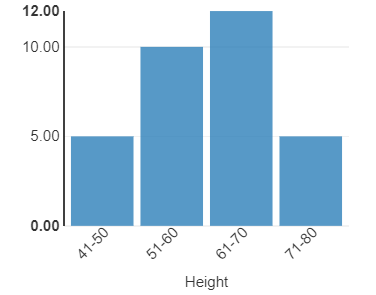### Pie chart

A pie chart displays the proportions of data items in comparison to each other. Each row in your data creates one slice of the pie.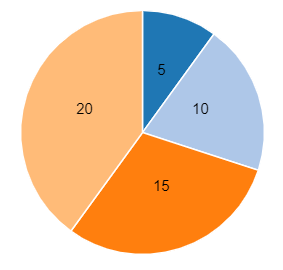Pie charts are useful for displaying categories of qualitative or descriptive nature.

### Donut chart

A donut chart also displays the proportions of data items in comparisons to each other, in the same manner that a pie chart does. Likewise, each row in your data creates on segment of the donut.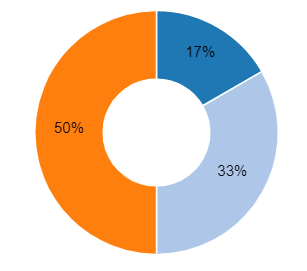### Scatterplot chart

A scatterplot chart plots two variables of a set of data on a graph. This is useful for visualizing any correlation between said variables.

### Column series chart

A column series chart is a bar chart. It creates series based on the columns of the data set; therefore, it is most appropriate for data sets with just a few columns.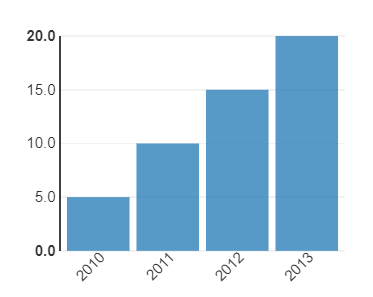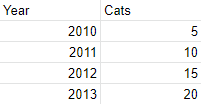Notice the orientation of the data; both quantities along the X and Y axes (years and number of cats) are columns in the data.  This is what distinguishes a column series chart from a row series chart - in a row series chart, both quantities would be rows in the data.

### Row series chart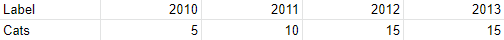While the visual chart is identical to a column series chart, now the x- and y-axes are taken from rows in the data.

When selecting between column and row series, make sure to consider in which direction your data is oriented.

### Column/row series [Line]

A column/row series [line] chart is a line chart; this is often used to visualize a trend in data over intervals of time.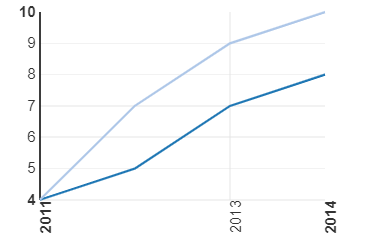As with regular column/row series charts, you can create either a column or a row series [line] based on the direction your data is oriented.

### Column/row series [Stack}

A column/row series [stack] chart is a stacked bar chart; this is often used to break down large categories and compare parts of the whole.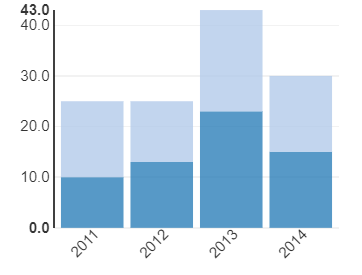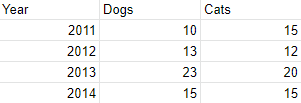As with the column/row series chart and column/row series chart [line], the values that display on the x and y-axes depend on the direction that your data is oriented.

## What values do you want on the X and Y axes?

There is currently no way to independently select the X and Y axes of your bar, line, and stack charts.

In order to select the values that will go onto the X and Y axes,  you must go into your data and determine whether you should use a column or row series chart.

Take the chart below for an example. Assume you want to display the `Year` column along the X axis, and the `Cats` column along the Y axis. Because you want to visualize columns, you would select Column Series Chart as the chart type in the editor.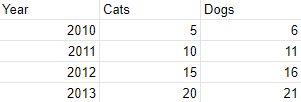## How many individual series on a chart do you want to draw?

This would depend on the number of columns or rows you have in your data. In the above data, you could display up to two series on the same chart.

For example, if you want to display dogs and cats on the Y axis, go to the Views pane, expand the view, and add any columns that you want to visualize on your graph using the Chart columns field.